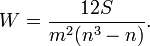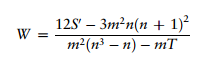# W Statistic (Coefficient of Concordance)

Statistics Definitions > W Statistic (Coefficient of Concordance)

## What is the W Statistic?

Kendall’s W statistic (sometimes called the Coefficient of Concordance) is a non parametric statistic used to assess agreement between different raters, and ranges from 0 to 1. Zero is no agreement at all between raters, while 1 is perfect agreement. The statistic is calculated either on an interval scale or on an ordinal scale.

For example, let’s say a group of workers were asked to rank a list of workplace concerns from least important to most important.

• If the W Statistic is 0, that means everyone ranked the list differently (or randomly).
• If the W Statistic is 1, then everyone ranked the list in exactly the same order.

Caution: you should only use the W statistic for measuring concordance between variables that are measuring the same general properties of objects. For example, if negative and positive correlations are of equal importance, this would not be a suitable test.

W is the notation used if the statistic is from a population. W̄ is the notation for the sample statistic.

## Formula

The formula for the W statistic is:Where:

• S is the sum of squared deviations,
• m is the number of judges (raters),
• n is the total number of objects being ranked.

A Second formula can also be used that gives the same results, where T is a correction factor for tied ranks:.

In theory, Kendall’s W can be used for 2 judges. However, Cohen’s Kappa or Spearman’s Rank Correlation Coefficient are better choices in this case.

According to Pierre Legendre, the term “judges” is usually only used in the social sciences. But Kendall’s W isn’t restricted to situations in the social sciences where people rank a list of items. For example, judges could be:

• Species (in community ecology), where population size can be used to evaluate habitat quality.
• Characteristics of different species, individuals, or populations (in taxonomy).

Reference:
Legendre, P. 2010. Coefficient of Concordance. Pp. 164-169 in: Encyclopedia of Research Design, Vol 1. NJ Salkind ed. SAGE Publications Inc.

CITE THIS AS:
Stephanie Glen. "W Statistic (Coefficient of Concordance)" From StatisticsHowTo.com: Elementary Statistics for the rest of us! https://www.statisticshowto.com/w-statistic/